Question

# A 35.4 kg mass attached to a spring oscillates with a period of 3.30 s. Find...

A 35.4 kg mass attached to a spring oscillates with a period of 3.30 s. Find the force constant of the spring.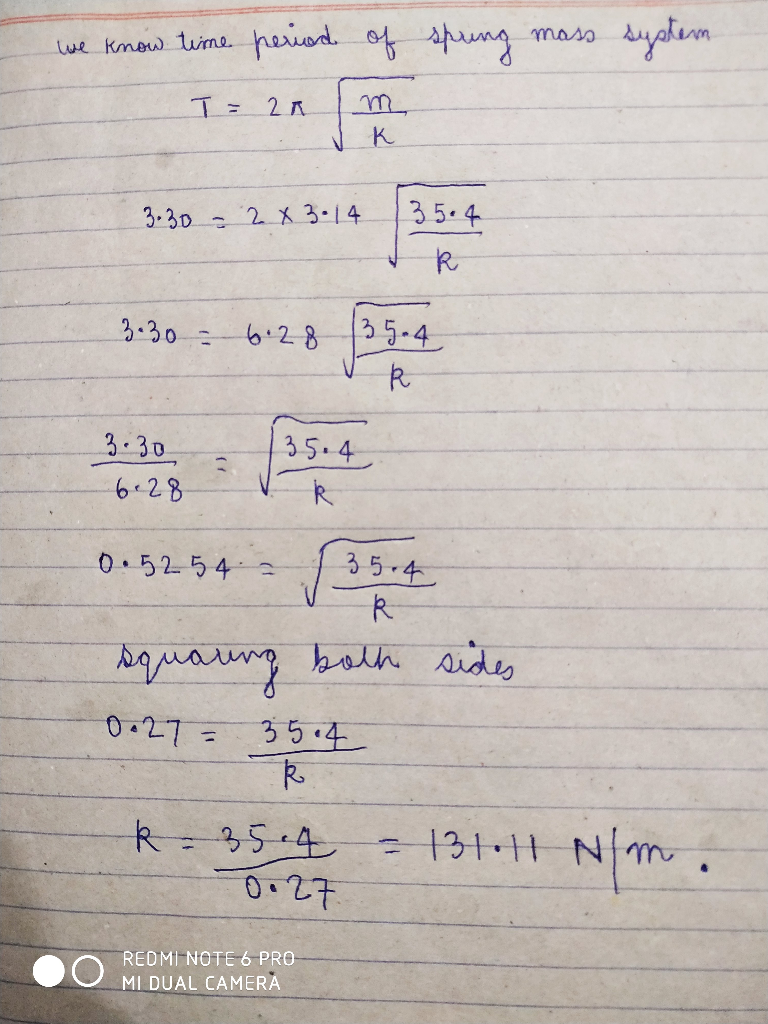please Rate me, In case of doubt feel free to ask.

#### Earn Coins

Coins can be redeemed for fabulous gifts.

Similar Homework Help Questions
• ### A mass attached to a spring oscillates with a period of 3.18 s. If the mass...

A mass attached to a spring oscillates with a period of 3.18 s. If the mass starts from rest at x = 0.0480 m and time t = 0, where is it at time t = 3.14 s?

• ### A 7 kg mass is attached to the end of a spring. The spring oscillates with...

A 7 kg mass is attached to the end of a spring. The spring oscillates with a frequency of 0.45 Hz. What is the spring constant of the spring?

• ### A mass attached to a spring oscillates with a period of 1.71 s and an amplitude...

A mass attached to a spring oscillates with a period of 1.71 s and an amplitude of 0.041 m. If the mass starts at x = 0.041 m at time t = 0, where is it at time t = 5.61 s

• ### A mass attached to a spring oscillates with a period of 3.15 s (a) If the...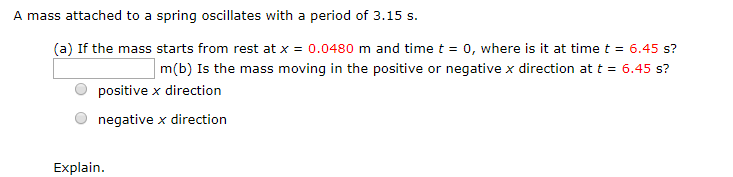A mass attached to a spring oscillates with a period of 3.15 s (a) If the mass starts from rest at x = 0.0480 m and time t = 0, where is it at time t = 6.45 s? m(b) Is the mass moving in the positive or negative x direction at t - 6.45 s? Opositive x direction O negative x direction Explain.

• ### A 0.500 kg mass is attached to a horizontal spring and oscillates such that its position...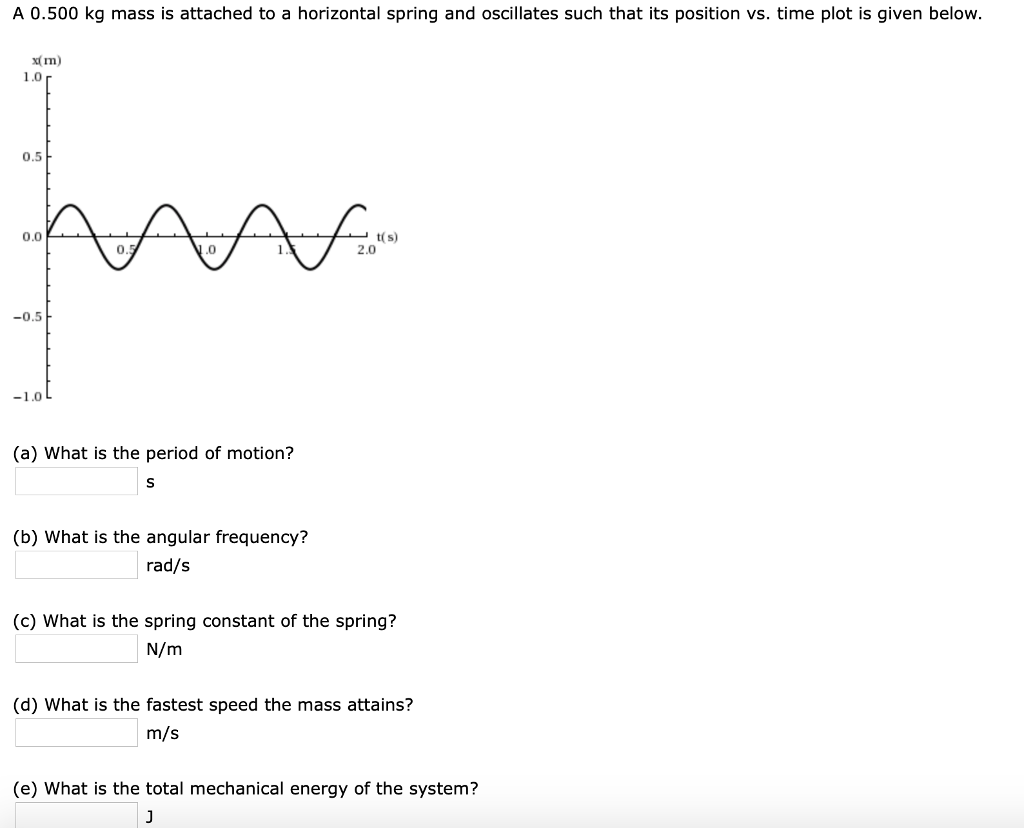A 0.500 kg mass is attached to a horizontal spring and oscillates such that its position vs. time plot is given below. x Im) 1.0 0.5 .0 2.0 -0.5 (a) What is the period of motion? (b) What is the angular frequency? rad/s (c) What is the spring constant of the spring? N/m (d) What is the fastest speed the mass attains? m/s (e) What is the total mechanical energy of the system?

• ### . A mass is attached to a spring. The position of the mass as it oscillates...

. A mass is attached to a spring. The position of the mass as it oscillates on the spring is given by: y = A cos (8.2t) where the value of t is in seconds and A is 6.2 cm. (a) What is the period of the oscillator? (2 pts) (b) What is the velocity of the oscillator at time t = 0 and at time t = T/4? Give magnitude and direction (+ or – y direction). (4 pts)...

• ### A 0.200 kg mass is attached to a horizontal spring and oscillates such that its position...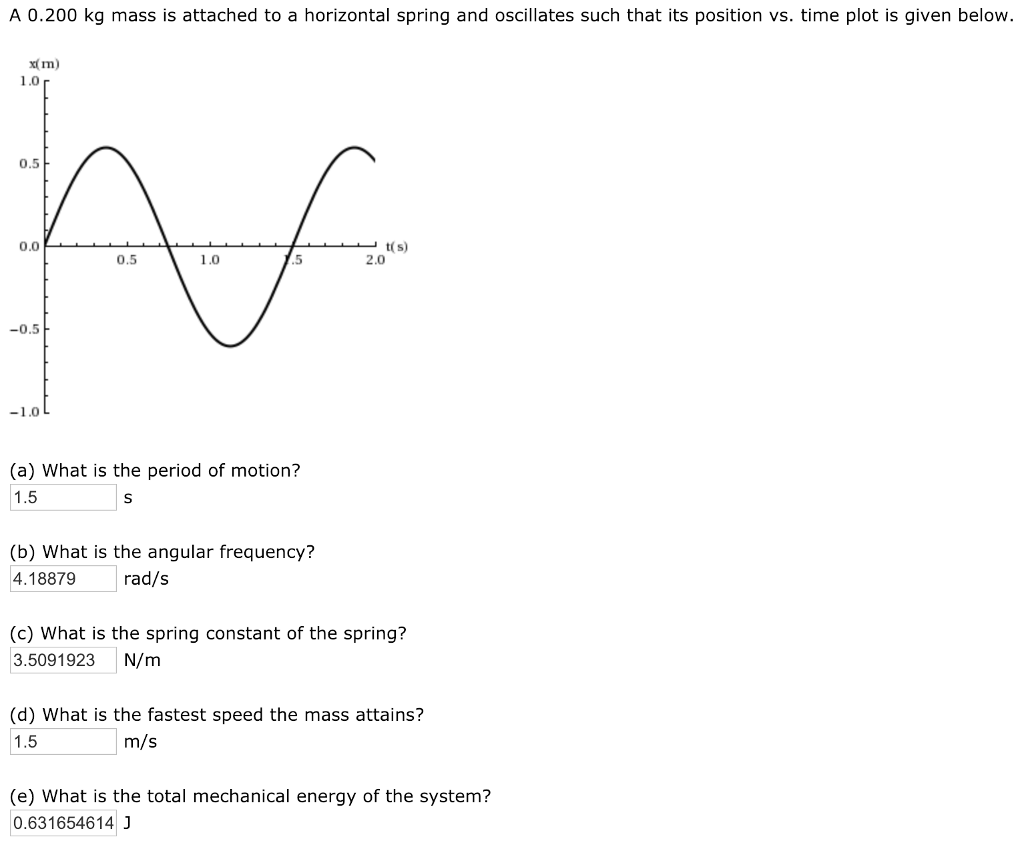A 0.200 kg mass is attached to a horizontal spring and oscillates such that its position vs. time plot is given below. x( Im) 1.0 r 0.5 0.0 0.5 1.0 .5 2.0 -0.5 -10L (a) What is the period of motion? 1.5 (b) What is the angular frequency? 4.18879rad/s (c) What is the spring constant of the spring? 3.5091923 N/m (d) What is the fastest speed the mass attains? 1.5 m/s (e) What is the total mechanical energy of the...

• ### A 0.490 kg mass suspended from a spring oscillates with a period of 1.50 s. How...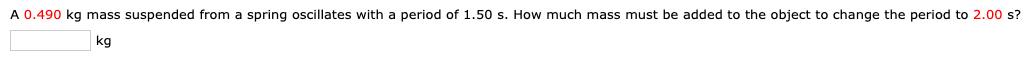A 0.490 kg mass suspended from a spring oscillates with a period of 1.50 s. How much mass must be added to the object to change the period to 2.00 s? kg

• ### 13b A 0.460 kg mass suspended from a spring oscillates with a period of 1.50 s....

13b A 0.460 kg mass suspended from a spring oscillates with a period of 1.50 s. How much mass must be added to the object to change the period to 2.00 s? ___ kg

• ### A 0.510 kg mass suspended from a spring oscillates with a period of 1.50 s. How...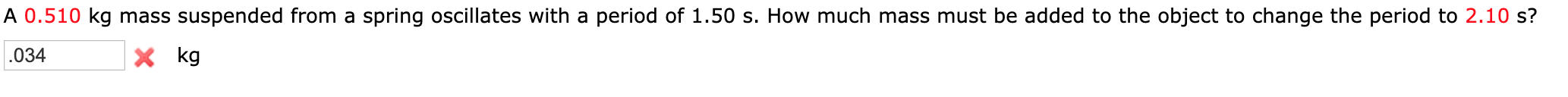A 0.510 kg mass suspended from a spring oscillates with a period of 1.50 s. How much mass must be added to the object to change the period to 2.10 s? x kg 034